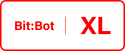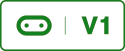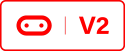# Line Following Robot (Arduino IDE)Go to https://bitbot.l33t.uk/arduino-ide/ultrasonic-example-arduino-ide for instructions on how to configure Arduino IDE for use with the BBC Micro:bit if you haven’t already. This code builds on the code found at https://bitbot.l33t.uk/arduino-ide/porting-bitbot-code-from-micropython-to-c

The ported code is below. It’s not a complete port I have added a clause when the robot sees 2 black lines it will go backwards. The method below was added to the ported code found here (under a new tab). Create a new tab and name it _01_LineFollower, select the new tab and paste the below code into the IDE.

Simple Line Follower
``/* * Simple line following algorithm for the 4Tronix Bit:Bot * Author David Bradshaw 2018 */void followLine(){    boolean isLeftLine = detectLine(0);  //Left line detector    boolean isRightLine = detectLine(1); //Right line detector    if(isLeftLine == true && isRightLine == true)    {        //both sensors can see a line        setColourRight(32,0,0);        setColourLeft(32,0,0);        while (isLeftLine == true && isRightLine == true)        {            isLeftLine = detectLine(0);  //Left line detector            isRightLine = detectLine(1); //Right line detector            backwards(125);        }        delay(350); //carry on going backwards for 350mS        forwards(0); //Stop bitbot    }       if (isLeftLine == true && isRightLine == false)    {        //Line on left hand side        setColourRight(0,32,0);        setColourLeft(32,0,0);        while (isLeftLine == true && isRightLine == false)        {            isLeftLine = detectLine(0);  //Left line detector            isRightLine = detectLine(1); //Right line detector            left(50);            delay(50);        }        forwards(0);    }    else if (isLeftLine == false && isRightLine == true)    {        //line on right hand side        setColourRight(32,0,0);        setColourLeft(0,32,0);        while (isLeftLine == false && isRightLine == true)        {            isLeftLine = detectLine(0);  //Left line detector            isRightLine = detectLine(1); //Right line detector            right(50);            delay(50);        }        forwards(0);    }    else if (isLeftLine == false && isRightLine == false)    {        //no line detected        setColourRight(0,32,0);        setColourLeft(0,32,0);        forwards(50);    }}``

I then added the following line in the void loop() method in the tab named _00_programLoop;

Call the above Function
``followLine();``

Now were using Arduino IDE we have freed up a lot of memory we can write a more complicated line following algorithm. The above algorithm is as simple as it gets for this stuff, in the near future I will add a much more complicated line follower algorithm.

You can download the full code from the resources section or by clicking Bitbot_Lib_LF.